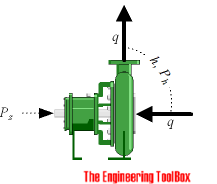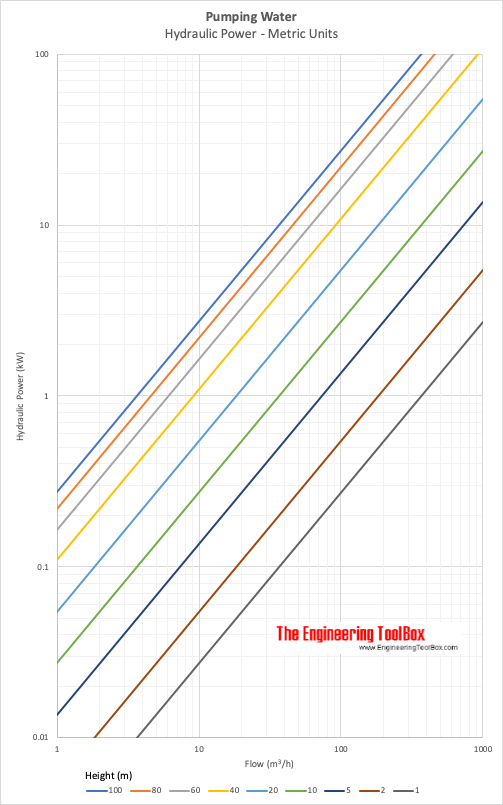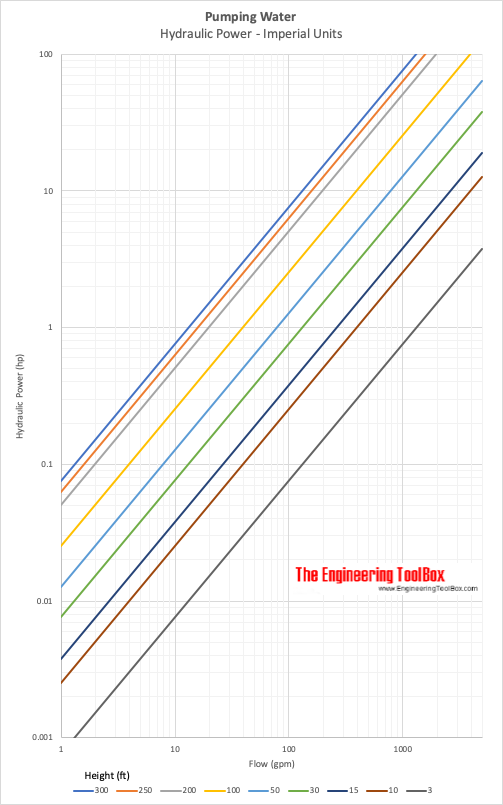Engineering ToolBox - Resources, Tools and Basic Information for Engineering and Design of Technical Applications!

# Pumps - Power Calculator

## Calculate pumps hydraulic and shaft power.

### Hydraulic Pump Power

The ideal hydraulic power to drive a pump depends on

• the mass flow rate the
• liquid density
• the differential height- either it is the static lift from one height to an other or the total head loss component of the system - and can be calculated like

Ph(kW) = q ρ g h / (3.6 106)

= q p / (3.6 106)                  (1)

where

Ph(kW) = hydraulic power (kW)

q = flow (m3/h)

ρ = density of fluid (kg/m3)

g = acceleration of gravity (9.81 m/s2)

p = differential pressure (N/m2, Pa)

The hydraulic Horse Power can be calculated as:

Ph(hp)Ph(kW) / 0.746                                  (2)

where

Ph(hp) = hydraulic horsepower (hp)

Or - alternatively

Ph(hp) = qgpm hft SG / (3960 η)                              (2b)

where

qgpm = flow (gpm)

SG = Specific Gravity (1 for water)

η = pump efficiency

### Example - Power pumping Water, SI Units

1 m3/h of water is pumped a head of 10 m. The theoretical pump power can be calculated as

Ph(kW) = (1 m3/h) (1000 kg/m3) (9.81 m/s2) (10 m) / (3.6 106)

= 0.027 kW

### Example - Power pumping Water, Imperial Units

600 gpm of water is pumped a head of 110 ft. The efficiency ofthe pump i s 60% (0.6) and the specific gravity of water is 1. The pump shaft power can be calculated as

Ph(kW) = (60 gpm) (110 ft) (1) / ((3960) (0.6))

= 27.8  hp

### Shaft Pump Power

The shaft power - the power required transferred from the motor to the shaft of the pump - depends on the efficiency of the pump and can be calculated as

Ps(kW) Ph(kW) / η                                     (3)

where

Ps(kW)  = shaft power (kW)

η = pump efficiency

### Online Pump Calculator - SI-units

The calculator below can used to calculate the hydraulic and shaft power of a pump:

q - flow (m3/h)

ρ - density of fluid (kg/m3)

g - gravity (m/s2)

η - pump efficiency

### Online Pump Calculator - Imperial units

The calculator below can used to calculate the hydraulic and shaft power of a pump using Imperial units:

q - flow capacity (gpm)

γ - specific weight of fluid (lb/ft3)

g - gravity (ft/s2)

η - pump efficiency

### Related Mobile Apps from The Engineering ToolBox

- free apps for offline use on mobile devices.## Related Topics

• Pumps - Piping systems and pumps - centrifugal pumps, displacement pumps - cavitation, viscosity, head and pressure, power consumption and more.

## Engineering ToolBox - SketchUp Extension - Online 3D modeling!

Add standard and customized parametric components - like flange beams, lumbers, piping, stairs and more - to your Sketchup model with the Engineering ToolBox - SketchUp Extension - enabled for use with the amazing, fun and free SketchUp Make and SketchUp Pro .Add the Engineering ToolBox extension to your SketchUp from the SketchUp Pro Sketchup Extension Warehouse!

Translate

## Privacy

We don't collect information from our users. Only emails and answers are saved in our archive. Cookies are only used in the browser to improve user experience.

Some of our calculators and applications let you save application data to your local computer. These applications will - due to browser restrictions - send data between your browser and our server. We don't save this data.

## Citation

• Engineering ToolBox, (2003). Pumps - Power Calculator. [online] Available at: https://www.engineeringtoolbox.com/pumps-power-d_505.html [Accessed Day Mo. Year].

Modify access date.

. .

#### Scientific Online Calculator3 30